No version for distro dashing. Known supported distros are highlighted in the buttons above.
No version for distro crystal. Known supported distros are highlighted in the buttons above.
No version for distro bouncy. Known supported distros are highlighted in the buttons above.

Package Summary

 Tags No category tags. Version 0.2.5 License BSD Build type CATKIN Use RECOMMENDED

Repository Summary

 Checkout URI https://github.com/locusrobotics/robot_navigation.git VCS Type git VCS Version tf2 Last Updated 2019-06-07 Dev Status DEVELOPED Released RELEASED

Package Description

Plugin based global planner implementing the nav_core2::GlobalPlanner interface.

Maintainers

• David V. Lu!!

dlux_global_planner

This package implements a plugin-based global wavefront planner that conforms to the nav_core2 interface. The two major components that you can plug-in are 1. PotentialCalculator - A function that calculates a numerical score (which we call potential) for some subset of the cells in the costmap. The potential should be zero at the goal and grow higher from there. 2. Traceback - A function that uses the potential to trace a path from the start position back to the goal position.

These are loaded via pluginlib to allow for maximal customizability. This package contains the wrapper code, whereas the dlux_plugins package provides a handful of implementations of the plugins.

History

This package is a refactoring for nav_core2 of the global_planner package which in turn was a refactoring of the navfn package. Much of the core implementation is based on design decisions of the authors of navfn, for better or for worse.

Weighing the Costmap

There is a fundamental tradeoff in planning between the length of the path and the costs contained within the costmap. This requires two variables. * The first is the neutral cost which is a penalty for moving from one cell to an adjacent cell (a.k.a. the cost for moving a distance equivalent to the grid resolution) * The second is the cost within the cell itself, which may be scaled up or down. Changing how these costs are used will result in possibly different paths. For example, low neutral costs will result in longer paths that avoid high costs. Higher neutral costs will sometimes result in paths that go through high costs if the path is much shorter.

The other key consideration in interpreting the costmap is whether to allow the planner to venture into cells marked as unknown in the costmap. There are three interpretations possible here. * LETHAL - Unknown cells are treated as lethal obstacles and cannot be passed through. * EXPENSIVE - Unknown cells are valid, but assigned a high cost. * FREE - Unknown cells are valid and given the same weight as free cells.

For convenience, the interpretation of all these costs is contained within the CostInterpreter class.

Potential Calculation

As mentioned above, the PotentialCalculator calculates a numerical score called potential for the cells in the costmap. This is stored in the PotentialGrid which is defined as a nav_grid.

using PotentialGrid = nav_grid::VectorNavGrid<float>;

The interface that must be implemented consists of up to two methods.

virtual void initialize(ros::NodeHandle& private_nh, nav_core2::Costmap::Ptr costmap,
CostInterpreter::Ptr cost_interpreter);
virtual void updatePotentials(PotentialGrid& potential_grid,
const geometry_msgs::Pose2D& start, const geometry_msgs::Pose2D& goal);

The first provides access to the Costmap/CostInterpreter. The second is where potentials are actually calculated. How the potentials are calculated is left to the plugin-writer, but in general, the potential should be zero at the goal and grow higher from there.

Traceback.

The Traceback uses the calculated potential to create a path from the start position back to the goal position. The interface to be implemented has two methods.

virtual void initialize(ros::NodeHandle& private_nh, CostInterpreter::Ptr cost_interpreter);
virtual nav_2d_msgs::Path2D getPath(const PotentialGrid& potential_grid,
const geometry_msgs::Pose2D& start, const geometry_msgs::Pose2D& goal,
double& path_cost);

The main thing to note is that the getPath method returns a Path2D and also calculates a path_cost, which is used when performing path caching.

Path Caching

Sometimes you don't want the global path to change if it doesn't have to. This global planner can cache its most recently returned plan (assuming the goal stays the same). Whether the old plan or new plan gets returned depends on a number of different factors. * If the old plan becomes invalid (navigates through an obstacle), the new plan should be used. * The relative path_cost of each of the plans. Generally, if the old plan is still valid, you should never get a new plan with a higher cost, but its possible depending on the exact plugin configuration.

There are four different configurations with regard to path caching, and when to use the cached path.

1. Don't ever use the cached path. This is the standard nav_core behavior and is enabled with path_caching=false.
2. Always use the cached plan if it is valid. It doesn't matter how much the new plan might improve the path, stay with the old path if it is valid. path_caching=true and improvement_threshold<0
3. Use the new plan if it is better than the cached plan. This will definitely rerun the planning algorithm each iteration, and use the new plan if its score according to the traceback is better at all than the old plan. path_caching=true and improvement_threshold=0. This is very close to configuration #1 but doesn't use new plans if their path cost is equal to or greater than the cached path cost.
4. Use the new plan if it is significantly better. Require the improvement to be greater than improvement_threshold to ensure plans that are minorly better aren't used. path_caching=true and improvement_threshold>=0

Base Parameters

• potential_calculator - default: dlux_plugins::AStar
• traceback - default: dlux_plugins::GradientPath
• publish_potential - default: false - Whether to publish the calculated potentials as an OccupancyGrid
• print_statistics - default: false - If true, will print the number of cells expanded, and the length and number of poses in the path.
• neutral_cost - default: 50 - see above section on weighing costmap
• scale - default: 3 - likewise
• unknown_interpretation - default: "expensive" - legal values: ["lethal", "expensive", "free"]
• path_caching - default: false
• improvement_threshold - default -1.0

The Kernel

One frequent operation that will be performed is to calculate the potential of a particular cell given the value of its neighboring cells. The most straightforward approach is to assign the potential as the minimum possible sum of a neighboring cell's potential and the cost of moving to that cell (i.e. the neutral cost plus the cost in the costmap). However, this approach does not take advantage of the two-dimensional structure of the grid. If there are two neighbors with previously calculated potentials, then we may want to combine them for a better potential.

This package provides the kernel_function class for combining neighboring potentials, which comes from navfn which in turn comes from A Light Formulation of the E Interpolated Path Replanner by Philippsen, Roland. Below, we provide a "brief" mathematical derivation of the calculation.

• For calculating the potential P for a cell X (a.k.a. P(X)) we will look at the four neighbors of the cell X, which we'll call A, B, C and D, where A and B are on the same axis (i.e. above and below X) and C and D are on the other axis.
• The cost of moving to a cell is the cost from the costmap, which we'll call h (to match the paper's notation)
• We assume, without loss of generality that
• P(A) <= P(B)
• P(C) <= P(D)
• P(A) <= P(C)
• If P(C) is infinite, that is, not initialized yet, the new potential calculation is straightforwardly .
• Otherwise, we want to find a value of P(X) that satisfies the equation
• It's possible there are no real values that satisfy the equation if in which case the straightforward update is used.
• Otherwise, through clever manipulation of the quadratic formula, we can solve the equation with the following:
• That all looks complicated, and computationally inefficient due to the square root operation. Hence, we calculate a second-degree Taylor series approximation as
• You can compare the two curves on this plot

It is this final approximation that we use in our potential calculation. Although these constants have been used since the origins of the nav stack, this is likely the first time the meaning of the constants has ever been documented.

Planner Node

This package also provides a standalone planner node, which will load a costmap from the global_costmap namespace, then listen on the /initialpose and /move_base_simple/goal topics for the start and goal poses respectively, and then publish the plan between the two poses as a Path and as Markers.

CHANGELOG
No CHANGELOG found.

Wiki Tutorials

See ROS Wiki Tutorials for more details.

Source Tutorials

Not currently indexed.

Launch files

No launch files found

Messages

No message files found.

Services

No service files found

Recent questions tagged dlux_global_planner at answers.ros.org

Package Summary

 Tags No category tags. Version 0.2.5 License BSD Build type CATKIN Use RECOMMENDED

Repository Summary

 Checkout URI https://github.com/locusrobotics/robot_navigation.git VCS Type git VCS Version master Last Updated 2019-06-24 Dev Status DEVELOPED Released RELEASED

Package Description

Plugin based global planner implementing the nav_core2::GlobalPlanner interface.

Maintainers

• David V. Lu!!

dlux_global_planner

This package implements a plugin-based global wavefront planner that conforms to the nav_core2 interface. The two major components that you can plug-in are 1. PotentialCalculator - A function that calculates a numerical score (which we call potential) for some subset of the cells in the costmap. The potential should be zero at the goal and grow higher from there. 2. Traceback - A function that uses the potential to trace a path from the start position back to the goal position.

These are loaded via pluginlib to allow for maximal customizability. This package contains the wrapper code, whereas the dlux_plugins package provides a handful of implementations of the plugins.

History

This package is a refactoring for nav_core2 of the global_planner package which in turn was a refactoring of the navfn package. Much of the core implementation is based on design decisions of the authors of navfn, for better or for worse.

Weighing the Costmap

There is a fundamental tradeoff in planning between the length of the path and the costs contained within the costmap. This requires two variables. * The first is the neutral cost which is a penalty for moving from one cell to an adjacent cell (a.k.a. the cost for moving a distance equivalent to the grid resolution) * The second is the cost within the cell itself, which may be scaled up or down. Changing how these costs are used will result in possibly different paths. For example, low neutral costs will result in longer paths that avoid high costs. Higher neutral costs will sometimes result in paths that go through high costs if the path is much shorter.

The other key consideration in interpreting the costmap is whether to allow the planner to venture into cells marked as unknown in the costmap. There are three interpretations possible here. * LETHAL - Unknown cells are treated as lethal obstacles and cannot be passed through. * EXPENSIVE - Unknown cells are valid, but assigned a high cost. * FREE - Unknown cells are valid and given the same weight as free cells.

For convenience, the interpretation of all these costs is contained within the CostInterpreter class.

Potential Calculation

As mentioned above, the PotentialCalculator calculates a numerical score called potential for the cells in the costmap. This is stored in the PotentialGrid which is defined as a nav_grid.

using PotentialGrid = nav_grid::VectorNavGrid<float>;

The interface that must be implemented consists of up to two methods.

virtual void initialize(ros::NodeHandle& private_nh, nav_core2::Costmap::Ptr costmap,
CostInterpreter::Ptr cost_interpreter);
virtual void updatePotentials(PotentialGrid& potential_grid,
const geometry_msgs::Pose2D& start, const geometry_msgs::Pose2D& goal);

The first provides access to the Costmap/CostInterpreter. The second is where potentials are actually calculated. How the potentials are calculated is left to the plugin-writer, but in general, the potential should be zero at the goal and grow higher from there.

Traceback.

The Traceback uses the calculated potential to create a path from the start position back to the goal position. The interface to be implemented has two methods.

virtual void initialize(ros::NodeHandle& private_nh, CostInterpreter::Ptr cost_interpreter);
virtual nav_2d_msgs::Path2D getPath(const PotentialGrid& potential_grid,
const geometry_msgs::Pose2D& start, const geometry_msgs::Pose2D& goal,
double& path_cost);

The main thing to note is that the getPath method returns a Path2D and also calculates a path_cost, which is used when performing path caching.

Path Caching

Sometimes you don't want the global path to change if it doesn't have to. This global planner can cache its most recently returned plan (assuming the goal stays the same). Whether the old plan or new plan gets returned depends on a number of different factors. * If the old plan becomes invalid (navigates through an obstacle), the new plan should be used. * The relative path_cost of each of the plans. Generally, if the old plan is still valid, you should never get a new plan with a higher cost, but its possible depending on the exact plugin configuration.

There are four different configurations with regard to path caching, and when to use the cached path.

1. Don't ever use the cached path. This is the standard nav_core behavior and is enabled with path_caching=false.
2. Always use the cached plan if it is valid. It doesn't matter how much the new plan might improve the path, stay with the old path if it is valid. path_caching=true and improvement_threshold<0
3. Use the new plan if it is better than the cached plan. This will definitely rerun the planning algorithm each iteration, and use the new plan if its score according to the traceback is better at all than the old plan. path_caching=true and improvement_threshold=0. This is very close to configuration #1 but doesn't use new plans if their path cost is equal to or greater than the cached path cost.
4. Use the new plan if it is significantly better. Require the improvement to be greater than improvement_threshold to ensure plans that are minorly better aren't used. path_caching=true and improvement_threshold>=0

Base Parameters

• potential_calculator - default: dlux_plugins::AStar
• traceback - default: dlux_plugins::GradientPath
• publish_potential - default: false - Whether to publish the calculated potentials as an OccupancyGrid
• print_statistics - default: false - If true, will print the number of cells expanded, and the length and number of poses in the path.
• neutral_cost - default: 50 - see above section on weighing costmap
• scale - default: 3 - likewise
• unknown_interpretation - default: "expensive" - legal values: ["lethal", "expensive", "free"]
• path_caching - default: false
• improvement_threshold - default -1.0

The Kernel

One frequent operation that will be performed is to calculate the potential of a particular cell given the value of its neighboring cells. The most straightforward approach is to assign the potential as the minimum possible sum of a neighboring cell's potential and the cost of moving to that cell (i.e. the neutral cost plus the cost in the costmap). However, this approach does not take advantage of the two-dimensional structure of the grid. If there are two neighbors with previously calculated potentials, then we may want to combine them for a better potential.

This package provides the kernel_function class for combining neighboring potentials, which comes from navfn which in turn comes from A Light Formulation of the E Interpolated Path Replanner by Philippsen, Roland. Below, we provide a "brief" mathematical derivation of the calculation.

• For calculating the potential P for a cell X (a.k.a. P(X)) we will look at the four neighbors of the cell X, which we'll call A, B, C and D, where A and B are on the same axis (i.e. above and below X) and C and D are on the other axis.
• The cost of moving to a cell is the cost from the costmap, which we'll call h (to match the paper's notation)
• We assume, without loss of generality that
•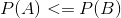•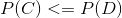•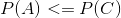• If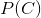is infinite, that is, not initialized yet, the new potential calculation is straightforwardly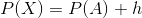.
• Otherwise, we want to find a value of P(X) that satisfies the equation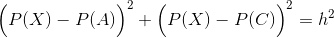• It's possible there are no real values that satisfy the equation if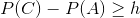in which case the straightforward update is used.
• Otherwise, through clever manipulation of the quadratic formula, we can solve the equation with the following:
•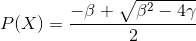•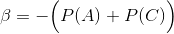•• That all looks complicated, and computationally inefficient due to the square root operation. Hence, we reformulate the equation in terms of a new variable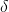and calculate a second-degree Taylor series approximation as
•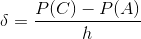•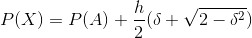•• If you're really interested, you can look into the full derivation
• You can compare these equations on this plot

It is this final approximation that we use in our potential calculation. Although this method has been used since the origins of the nav stack, this is likely the first time the meaning of the constants has ever been documented.

Planner Node

This package also provides a standalone planner node, which will load a costmap from the global_costmap namespace, then listen on the /initialpose and /move_base_simple/goal topics for the start and goal poses respectively, and then publish the plan between the two poses as a Path and as Markers.

CHANGELOG
No CHANGELOG found.

Wiki Tutorials

See ROS Wiki Tutorials for more details.

Source Tutorials

Not currently indexed.

Launch files

No launch files found

Messages

No message files found.

Services

No service files found

Recent questions tagged dlux_global_planner at answers.ros.org

No version for distro ardent. Known supported distros are highlighted in the buttons above.

Package Summary

 Tags No category tags. Version 0.2.5 License BSD Build type CATKIN Use RECOMMENDED

Repository Summary

 Checkout URI https://github.com/locusrobotics/robot_navigation.git VCS Type git VCS Version master Last Updated 2019-06-24 Dev Status DEVELOPED Released RELEASED

Package Description

Plugin based global planner implementing the nav_core2::GlobalPlanner interface.

Maintainers

• David V. Lu!!

dlux_global_planner

This package implements a plugin-based global wavefront planner that conforms to the nav_core2 interface. The two major components that you can plug-in are 1. PotentialCalculator - A function that calculates a numerical score (which we call potential) for some subset of the cells in the costmap. The potential should be zero at the goal and grow higher from there. 2. Traceback - A function that uses the potential to trace a path from the start position back to the goal position.

These are loaded via pluginlib to allow for maximal customizability. This package contains the wrapper code, whereas the dlux_plugins package provides a handful of implementations of the plugins.

History

This package is a refactoring for nav_core2 of the global_planner package which in turn was a refactoring of the navfn package. Much of the core implementation is based on design decisions of the authors of navfn, for better or for worse.

Weighing the Costmap

There is a fundamental tradeoff in planning between the length of the path and the costs contained within the costmap. This requires two variables. * The first is the neutral cost which is a penalty for moving from one cell to an adjacent cell (a.k.a. the cost for moving a distance equivalent to the grid resolution) * The second is the cost within the cell itself, which may be scaled up or down. Changing how these costs are used will result in possibly different paths. For example, low neutral costs will result in longer paths that avoid high costs. Higher neutral costs will sometimes result in paths that go through high costs if the path is much shorter.

The other key consideration in interpreting the costmap is whether to allow the planner to venture into cells marked as unknown in the costmap. There are three interpretations possible here. * LETHAL - Unknown cells are treated as lethal obstacles and cannot be passed through. * EXPENSIVE - Unknown cells are valid, but assigned a high cost. * FREE - Unknown cells are valid and given the same weight as free cells.

For convenience, the interpretation of all these costs is contained within the CostInterpreter class.

Potential Calculation

As mentioned above, the PotentialCalculator calculates a numerical score called potential for the cells in the costmap. This is stored in the PotentialGrid which is defined as a nav_grid.

using PotentialGrid = nav_grid::VectorNavGrid<float>;

The interface that must be implemented consists of up to two methods.

virtual void initialize(ros::NodeHandle& private_nh, nav_core2::Costmap::Ptr costmap,
CostInterpreter::Ptr cost_interpreter);
virtual void updatePotentials(PotentialGrid& potential_grid,
const geometry_msgs::Pose2D& start, const geometry_msgs::Pose2D& goal);

The first provides access to the Costmap/CostInterpreter. The second is where potentials are actually calculated. How the potentials are calculated is left to the plugin-writer, but in general, the potential should be zero at the goal and grow higher from there.

Traceback.

The Traceback uses the calculated potential to create a path from the start position back to the goal position. The interface to be implemented has two methods.

virtual void initialize(ros::NodeHandle& private_nh, CostInterpreter::Ptr cost_interpreter);
virtual nav_2d_msgs::Path2D getPath(const PotentialGrid& potential_grid,
const geometry_msgs::Pose2D& start, const geometry_msgs::Pose2D& goal,
double& path_cost);

The main thing to note is that the getPath method returns a Path2D and also calculates a path_cost, which is used when performing path caching.

Path Caching

Sometimes you don't want the global path to change if it doesn't have to. This global planner can cache its most recently returned plan (assuming the goal stays the same). Whether the old plan or new plan gets returned depends on a number of different factors. * If the old plan becomes invalid (navigates through an obstacle), the new plan should be used. * The relative path_cost of each of the plans. Generally, if the old plan is still valid, you should never get a new plan with a higher cost, but its possible depending on the exact plugin configuration.

There are four different configurations with regard to path caching, and when to use the cached path.

1. Don't ever use the cached path. This is the standard nav_core behavior and is enabled with path_caching=false.
2. Always use the cached plan if it is valid. It doesn't matter how much the new plan might improve the path, stay with the old path if it is valid. path_caching=true and improvement_threshold<0
3. Use the new plan if it is better than the cached plan. This will definitely rerun the planning algorithm each iteration, and use the new plan if its score according to the traceback is better at all than the old plan. path_caching=true and improvement_threshold=0. This is very close to configuration #1 but doesn't use new plans if their path cost is equal to or greater than the cached path cost.
4. Use the new plan if it is significantly better. Require the improvement to be greater than improvement_threshold to ensure plans that are minorly better aren't used. path_caching=true and improvement_threshold>=0

Base Parameters

• potential_calculator - default: dlux_plugins::AStar
• traceback - default: dlux_plugins::GradientPath
• publish_potential - default: false - Whether to publish the calculated potentials as an OccupancyGrid
• print_statistics - default: false - If true, will print the number of cells expanded, and the length and number of poses in the path.
• neutral_cost - default: 50 - see above section on weighing costmap
• scale - default: 3 - likewise
• unknown_interpretation - default: "expensive" - legal values: ["lethal", "expensive", "free"]
• path_caching - default: false
• improvement_threshold - default -1.0

The Kernel

One frequent operation that will be performed is to calculate the potential of a particular cell given the value of its neighboring cells. The most straightforward approach is to assign the potential as the minimum possible sum of a neighboring cell's potential and the cost of moving to that cell (i.e. the neutral cost plus the cost in the costmap). However, this approach does not take advantage of the two-dimensional structure of the grid. If there are two neighbors with previously calculated potentials, then we may want to combine them for a better potential.

This package provides the kernel_function class for combining neighboring potentials, which comes from navfn which in turn comes from A Light Formulation of the E Interpolated Path Replanner by Philippsen, Roland. Below, we provide a "brief" mathematical derivation of the calculation.

• For calculating the potential P for a cell X (a.k.a. P(X)) we will look at the four neighbors of the cell X, which we'll call A, B, C and D, where A and B are on the same axis (i.e. above and below X) and C and D are on the other axis.
• The cost of moving to a cell is the cost from the costmap, which we'll call h (to match the paper's notation)
• We assume, without loss of generality that
•••• Ifis infinite, that is, not initialized yet, the new potential calculation is straightforwardly.
• Otherwise, we want to find a value of P(X) that satisfies the equation• It's possible there are no real values that satisfy the equation ifin which case the straightforward update is used.
• Otherwise, through clever manipulation of the quadratic formula, we can solve the equation with the following:
•••• That all looks complicated, and computationally inefficient due to the square root operation. Hence, we reformulate the equation in terms of a new variableand calculate a second-degree Taylor series approximation as
•••• If you're really interested, you can look into the full derivation
• You can compare these equations on this plot

It is this final approximation that we use in our potential calculation. Although this method has been used since the origins of the nav stack, this is likely the first time the meaning of the constants has ever been documented.

Planner Node

This package also provides a standalone planner node, which will load a costmap from the global_costmap namespace, then listen on the /initialpose and /move_base_simple/goal topics for the start and goal poses respectively, and then publish the plan between the two poses as a Path and as Markers.

CHANGELOG
No CHANGELOG found.

Wiki Tutorials

See ROS Wiki Tutorials for more details.

Source Tutorials

Not currently indexed.

Launch files

No launch files found

Messages

No message files found.

Services

No service files found

Recent questions tagged dlux_global_planner at answers.ros.org

No version for distro jade. Known supported distros are highlighted in the buttons above.

Package Summary

 Tags No category tags. Version 0.2.5 License BSD Build type CATKIN Use RECOMMENDED

Repository Summary

 Checkout URI https://github.com/locusrobotics/robot_navigation.git VCS Type git VCS Version master Last Updated 2019-06-24 Dev Status DEVELOPED Released RELEASED

Package Description

Plugin based global planner implementing the nav_core2::GlobalPlanner interface.

Maintainers

• David V. Lu!!

dlux_global_planner

This package implements a plugin-based global wavefront planner that conforms to the nav_core2 interface. The two major components that you can plug-in are 1. PotentialCalculator - A function that calculates a numerical score (which we call potential) for some subset of the cells in the costmap. The potential should be zero at the goal and grow higher from there. 2. Traceback - A function that uses the potential to trace a path from the start position back to the goal position.

These are loaded via pluginlib to allow for maximal customizability. This package contains the wrapper code, whereas the dlux_plugins package provides a handful of implementations of the plugins.

History

This package is a refactoring for nav_core2 of the global_planner package which in turn was a refactoring of the navfn package. Much of the core implementation is based on design decisions of the authors of navfn, for better or for worse.

Weighing the Costmap

There is a fundamental tradeoff in planning between the length of the path and the costs contained within the costmap. This requires two variables. * The first is the neutral cost which is a penalty for moving from one cell to an adjacent cell (a.k.a. the cost for moving a distance equivalent to the grid resolution) * The second is the cost within the cell itself, which may be scaled up or down. Changing how these costs are used will result in possibly different paths. For example, low neutral costs will result in longer paths that avoid high costs. Higher neutral costs will sometimes result in paths that go through high costs if the path is much shorter.

The other key consideration in interpreting the costmap is whether to allow the planner to venture into cells marked as unknown in the costmap. There are three interpretations possible here. * LETHAL - Unknown cells are treated as lethal obstacles and cannot be passed through. * EXPENSIVE - Unknown cells are valid, but assigned a high cost. * FREE - Unknown cells are valid and given the same weight as free cells.

For convenience, the interpretation of all these costs is contained within the CostInterpreter class.

Potential Calculation

As mentioned above, the PotentialCalculator calculates a numerical score called potential for the cells in the costmap. This is stored in the PotentialGrid which is defined as a nav_grid.

using PotentialGrid = nav_grid::VectorNavGrid<float>;

The interface that must be implemented consists of up to two methods.

virtual void initialize(ros::NodeHandle& private_nh, nav_core2::Costmap::Ptr costmap,
CostInterpreter::Ptr cost_interpreter);
virtual void updatePotentials(PotentialGrid& potential_grid,
const geometry_msgs::Pose2D& start, const geometry_msgs::Pose2D& goal);

The first provides access to the Costmap/CostInterpreter. The second is where potentials are actually calculated. How the potentials are calculated is left to the plugin-writer, but in general, the potential should be zero at the goal and grow higher from there.

Traceback.

The Traceback uses the calculated potential to create a path from the start position back to the goal position. The interface to be implemented has two methods.

virtual void initialize(ros::NodeHandle& private_nh, CostInterpreter::Ptr cost_interpreter);
virtual nav_2d_msgs::Path2D getPath(const PotentialGrid& potential_grid,
const geometry_msgs::Pose2D& start, const geometry_msgs::Pose2D& goal,
double& path_cost);

The main thing to note is that the getPath method returns a Path2D and also calculates a path_cost, which is used when performing path caching.

Path Caching

Sometimes you don't want the global path to change if it doesn't have to. This global planner can cache its most recently returned plan (assuming the goal stays the same). Whether the old plan or new plan gets returned depends on a number of different factors. * If the old plan becomes invalid (navigates through an obstacle), the new plan should be used. * The relative path_cost of each of the plans. Generally, if the old plan is still valid, you should never get a new plan with a higher cost, but its possible depending on the exact plugin configuration.

There are four different configurations with regard to path caching, and when to use the cached path.

1. Don't ever use the cached path. This is the standard nav_core behavior and is enabled with path_caching=false.
2. Always use the cached plan if it is valid. It doesn't matter how much the new plan might improve the path, stay with the old path if it is valid. path_caching=true and improvement_threshold<0
3. Use the new plan if it is better than the cached plan. This will definitely rerun the planning algorithm each iteration, and use the new plan if its score according to the traceback is better at all than the old plan. path_caching=true and improvement_threshold=0. This is very close to configuration #1 but doesn't use new plans if their path cost is equal to or greater than the cached path cost.
4. Use the new plan if it is significantly better. Require the improvement to be greater than improvement_threshold to ensure plans that are minorly better aren't used. path_caching=true and improvement_threshold>=0

Base Parameters

• potential_calculator - default: dlux_plugins::AStar
• traceback - default: dlux_plugins::GradientPath
• publish_potential - default: false - Whether to publish the calculated potentials as an OccupancyGrid
• print_statistics - default: false - If true, will print the number of cells expanded, and the length and number of poses in the path.
• neutral_cost - default: 50 - see above section on weighing costmap
• scale - default: 3 - likewise
• unknown_interpretation - default: "expensive" - legal values: ["lethal", "expensive", "free"]
• path_caching - default: false
• improvement_threshold - default -1.0

The Kernel

One frequent operation that will be performed is to calculate the potential of a particular cell given the value of its neighboring cells. The most straightforward approach is to assign the potential as the minimum possible sum of a neighboring cell's potential and the cost of moving to that cell (i.e. the neutral cost plus the cost in the costmap). However, this approach does not take advantage of the two-dimensional structure of the grid. If there are two neighbors with previously calculated potentials, then we may want to combine them for a better potential.

This package provides the kernel_function class for combining neighboring potentials, which comes from navfn which in turn comes from A Light Formulation of the E Interpolated Path Replanner by Philippsen, Roland. Below, we provide a "brief" mathematical derivation of the calculation.

• For calculating the potential P for a cell X (a.k.a. P(X)) we will look at the four neighbors of the cell X, which we'll call A, B, C and D, where A and B are on the same axis (i.e. above and below X) and C and D are on the other axis.
• The cost of moving to a cell is the cost from the costmap, which we'll call h (to match the paper's notation)
• We assume, without loss of generality that
•••• Ifis infinite, that is, not initialized yet, the new potential calculation is straightforwardly.
• Otherwise, we want to find a value of P(X) that satisfies the equation• It's possible there are no real values that satisfy the equation ifin which case the straightforward update is used.
• Otherwise, through clever manipulation of the quadratic formula, we can solve the equation with the following:
•••• That all looks complicated, and computationally inefficient due to the square root operation. Hence, we reformulate the equation in terms of a new variableand calculate a second-degree Taylor series approximation as
•••• If you're really interested, you can look into the full derivation
• You can compare these equations on this plot

It is this final approximation that we use in our potential calculation. Although this method has been used since the origins of the nav stack, this is likely the first time the meaning of the constants has ever been documented.

Planner Node

This package also provides a standalone planner node, which will load a costmap from the global_costmap namespace, then listen on the /initialpose and /move_base_simple/goal topics for the start and goal poses respectively, and then publish the plan between the two poses as a Path and as Markers.

CHANGELOG
No CHANGELOG found.

Wiki Tutorials

See ROS Wiki Tutorials for more details.

Source Tutorials

Not currently indexed.

Launch files

No launch files found

Messages

No message files found.

Services

No service files found

Recent questions tagged dlux_global_planner at answers.ros.org

No version for distro hydro. Known supported distros are highlighted in the buttons above.